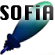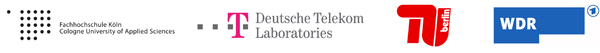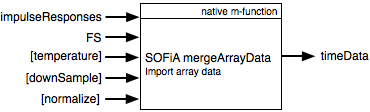Welcome to SOFiA
Who is behind SOFiA
Feature overview
System overview
Function reference
mergeArrayData
F/D/T
gauss
lebedev
S/W/G
S/T/C
W/G/C
S/F/E
M/F
R/F/I
P/D/C
I/T/C
makeMTX
makeIR
visual3D
Coordinate System
Application Examples
Example 1
Example 2
Example 3
Example 4
Example 5
Example 6
Example 7
Example 8
Array Datasets
VariSphear system
Groups and Mailinglists
Contact and Support
How to Reference

# Import array dataset

The function mergeArrayData() merges array measurement data to structs that can be read by the SOFiA F/D/T frequency domain transform. The mergeArrayData() function allows to downsample and normalize the impulse responses.### ARGUMENTS

Input

Name Type Purpose Default
impulseResponses float mtx Impulse Response Data
Rows: IR-Data
Cols: Different positions
-
FS int Sampling Frequency -
temperature float Air Temperature in °C 20
downSample int Downsample Factor 1
normalize int Normalization Flag 1:on 0:off 1

Output

Name Type Purpose Default
timeData struct Time domain Data -

The structs contain the following fields:

 Field Type Purpose .impulseResponses float mtx Impulse Response Data N:Channels, M:Samples .FS int Sampling Frequency .downSample int Downsampling Factor (from Input) .averageAirTemp float Average air temperature .irOverlay float vector Overlay of all impulse responses (PLOT)

### SOME DETAILS

The downsampling is done using DECIMATE and a FIR low pass filter of order 30. See MATLAB documentation for more information.
(MATLAB Signal Processing Library required for downsampling.)

The normalization is done with respect to the highest overall value found in the complete session.

## FILE(S):

 File Type OS/Matlab sofia_mergeArrayData.m Help header, Fuction All OS

 `/*timeData = sofia_mergeArrayData(impulseResponses, FS, temperature,...` `[downsample], [normalized])` `------------------------------------------------------------------------------------` `timeData` ```Struct with fileds: .impulseResponses``` `.FS` `.downSample` `.averageAirTemp` `.irOverlay` ``` [N:Channes M:Samples]```     `Temperature in DEG` `Plot this for a good total` `overview if the dataset` `------------------------------------------------------------------------------------` `impulseResponse` ```Impulse response data [M:Channels N:Samples] !The audio data must be in in columns as it is usual in MATLAB. But SOFiA works on audio data in rows as it is usual in audio editors etc. Therefore the output IR matrix is flipped over.``` `FS` `Sampling frequency of the source material in 1/s` `radius` `Array sphere radius in meters` `temperature` ```Average air temperature during the captureThis is important for F/D/T to calculate the kr-vector. (This value is simply passed thru).``` `downSample` ```Downsampling factor [default = 1: No downsampling] Downsampling is done using DECIMATE and a FIR low pass filter of order 30. See MATLAB documentation for more information. !!! MATLAB Signal Processing Library required``` `normalize` ```Normalize flag 1:on, 0:off [default = 1: on] Normalizes the impulse response with respect to the absolute maximum value within the complete dataset.``` `directory` ```VSA dataset directory. If not definded a user dialog opens to pick a directory``` `*/`